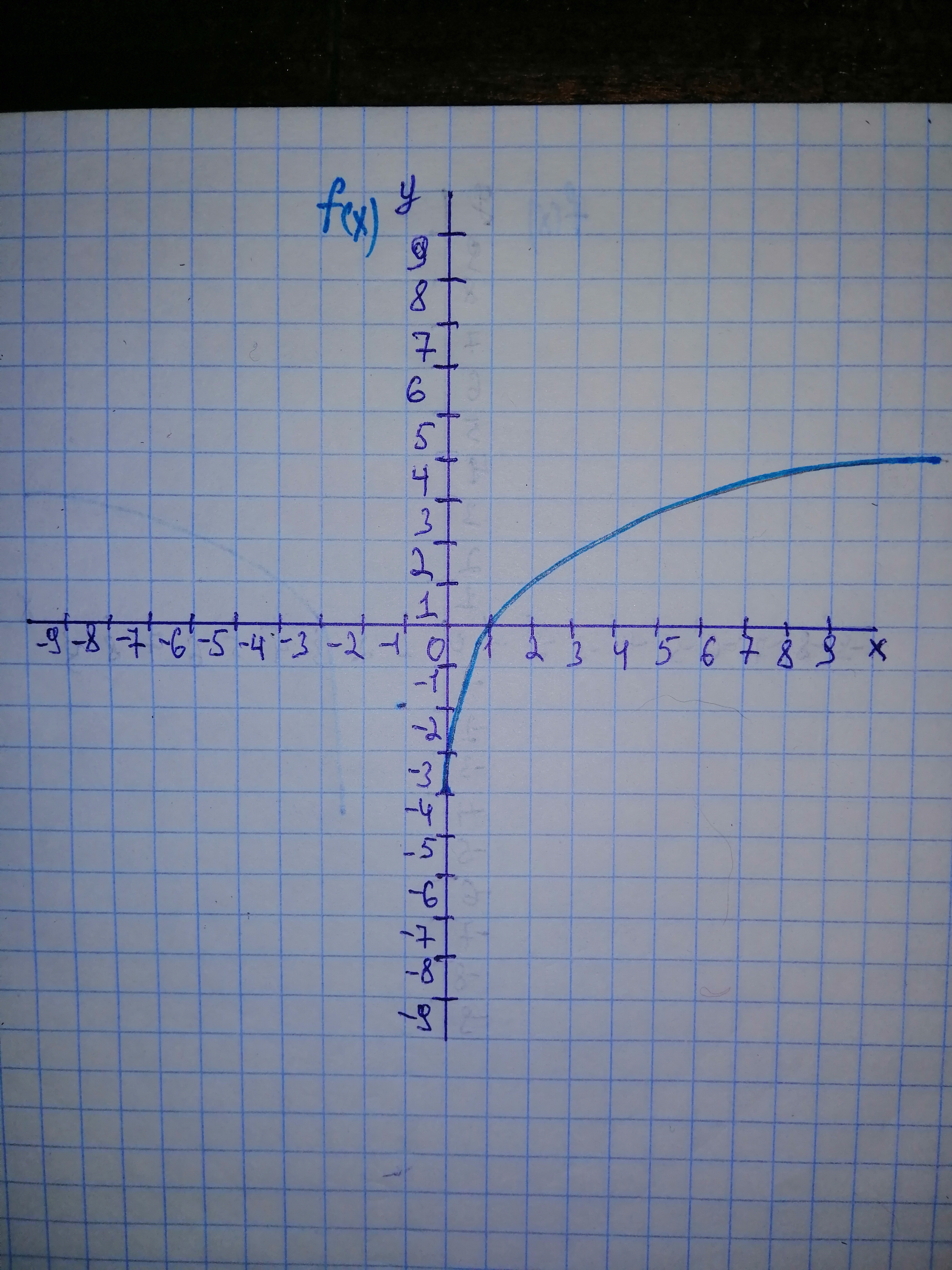# Begin by graphing f(x)= log_{2}x Then use transformations of this graph to graph the given function. What is the graph's x-intercept? What is the vertical asymptote? Use the graphs to determine each function's domain and range. g(x)= log_{2}(x - 2)Daniaal Sanchez 2021-02-21 Answered
Begin by graphing Then use transformations of this graph to graph the given function. What is the graphs
You can still ask an expert for help

• Questions are typically answered in as fast as 30 minutes

Solve your problem for the price of one coffee

• Math expert for every subject
• Pay only if we can solve itArham Warner

Step 1 Graph ofStep 2 Graph of From ${\mathrm{log}}_{2}x$ the graph is translated to the right 2 units. The x-intercept is (3, 0). There is a vertical asymptote at $x=2$. The domain of the graph is . The range is all real numbers.